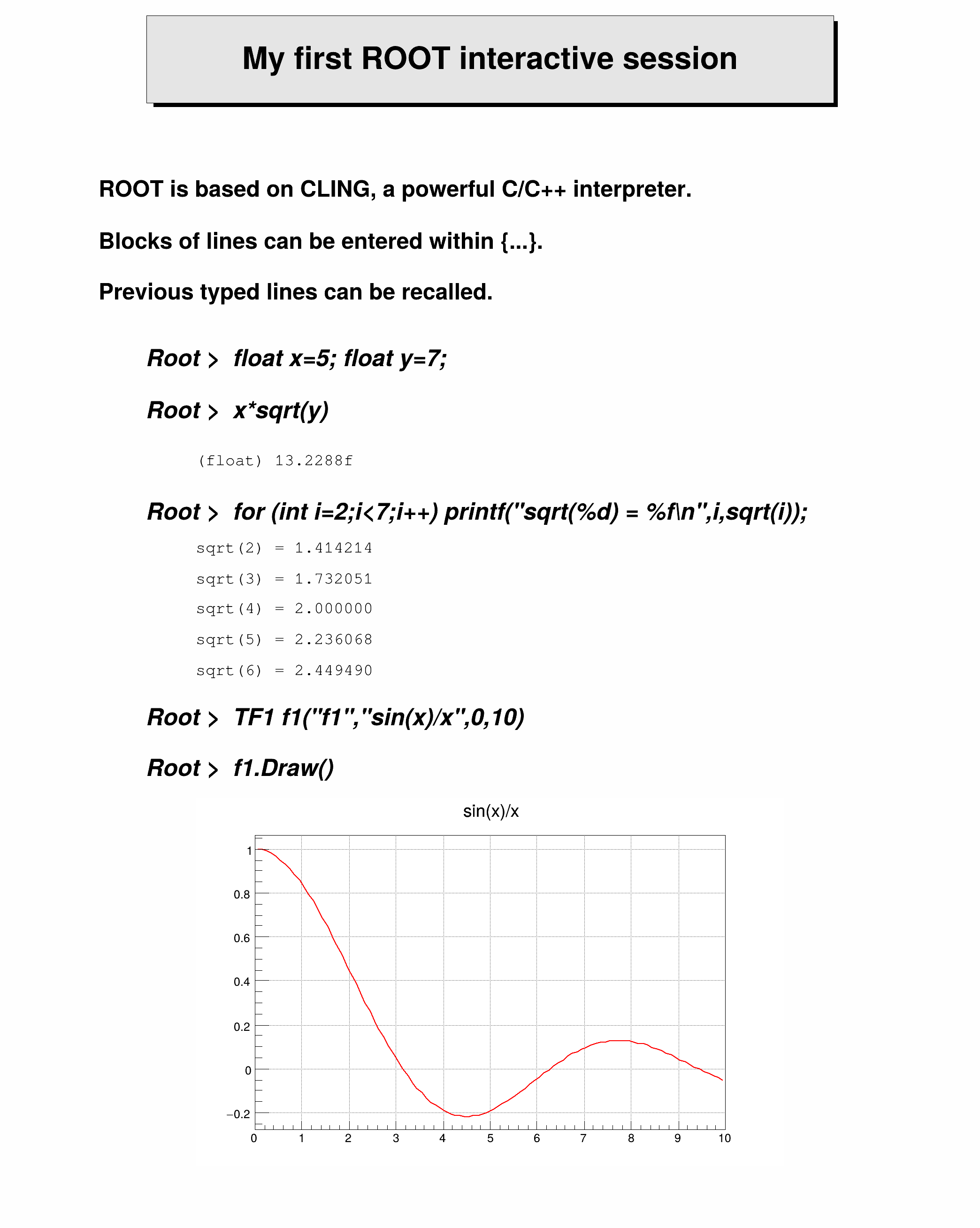ROOT   Reference Guidefirst.C File Reference

## Detailed Descriptionvoid first() {
TCanvas *nut = new TCanvas("nut", "FirstSession",100,10,700,900);
nut->Range(0,0,20,24);
nut->SetFillColor(10);
nut->SetBorderSize(2);
TPaveLabel *pl = new TPaveLabel(3,22,17,23.7,
"My first ROOT interactive session","br");
pl->SetFillColor(18);
pl->Draw();
TText t(0,0,"a");
t.SetTextFont(62);
t.SetTextSize(0.025);
t.SetTextAlign(12);
t.DrawText(2,20.3,"ROOT is based on CINT, a powerful C/C++ interpreter.");
t.DrawText(2,19.3,"Blocks of lines can be entered within {...}.");
t.DrawText(2,18.3,"Previous typed lines can be recalled.");
t.SetTextFont(72);
t.SetTextSize(0.026);
t.DrawText(3,17,"Root > float x=5; float y=7;");
t.DrawText(3,16,"Root > x*sqrt(y)");
t.DrawText(3,14,
"Root > for (int i=2;i<7;i++) printf(\"sqrt(%d) = %f\\n\",i,sqrt(i));");
t.DrawText(3,10,"Root > TF1 f1(\"f1\",\"sin(x)/x\",0,10)");
t.DrawText(3, 9,"Root > f1.Draw()");
t.SetTextFont(81);
t.SetTextSize(0.018);
t.DrawText(4,15,"(float) 13.2288f");
t.DrawText(4,13.3,"sqrt(2) = 1.414214");
t.DrawText(4,12.7,"sqrt(3) = 1.732051");
t.DrawText(4,12.1,"sqrt(4) = 2.000000");
t.DrawText(4,11.5,"sqrt(5) = 2.236068");
t.DrawText(4,10.9,"sqrt(6) = 2.449490");
TF1 *f1 = new TF1("f1","sin(x)/x",0,10);
f1->Draw();
}
virtual void SetFillColor(Color_t fcolor)
Set the fill area color.
Definition: TAttFill.h:37
The Canvas class.
Definition: TCanvas.h:23
1-Dim function class
Definition: TF1.h:213
void Draw(Option_t *option="") override
Draw this function with its current attributes.
Definition: TF1.cxx:1347
The most important graphics class in the ROOT system.
void SetBorderSize(Short_t bordersize) override
void SetGrid(Int_t valuex=1, Int_t valuey=1) override
void Range(Double_t x1, Double_t y1, Double_t x2, Double_t y2) override
Set world coordinate system for the pad.
void Draw(Option_t *option="") override